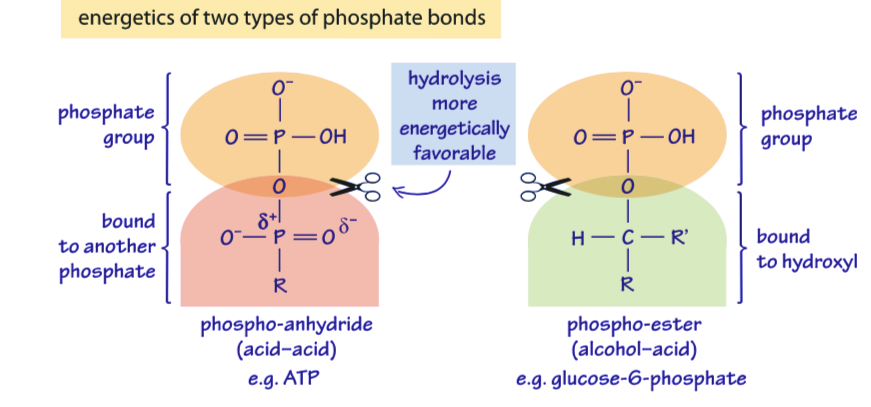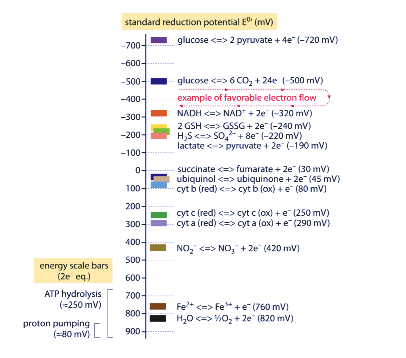## Entropy Supply Entropy Production and Free Energy

The free energy is defined as available energy . The question is how this is related to the entropy production. If we assume that supply of entropy is primarily enthalpic in nature we can write:

1. $\Delta S_e = \frac{\Delta H}{T} (eq.1)$ The free energy relation can be expressed as:

2. $\Delta G = \Delta H - T \Delta S (eq.2)$ Using equations (1) and (2) we have,

3. $\Delta S = \Delta S_e - \frac{\Delta G}{T}$ From our previous discussions we know that for open systems the book keeping of entropy follows from :

4. $\Delta S = \Delta S_e + \Delta S_i (eq.3)$

The equation (4) and (2) imply,

1. $T\Delta S_i = -\Delta G \ge 0 (eq.4)$

It thus follows that second law implies that the dissipation is greater than zero that also implies teh negative nature of the overall free energy change for a syatem.

## Points to note

• The second law as per Boltzman description is expressed in terms of positive changes in entropy ($$\Delta S_i>0$$)
• The free energy changes is known to be negative ($$\Delta G\le 0$$)
• The two relations, one minimizing and the other maximizing, are thus consistent and equivalent.

## Is free energy an equilibrium concept?

Let is consider a chemical reaction (@simple1) $A =B (eq.5)$

The simpliest way of writing free energy is

1. $\Delta G = \mu_B - \mu_A (eq.6)$ where, $$\mu$$ is the chemical potential and is given by,
2. $\mu = \mu_o + RT ln C (eq.7)$ Essentially this formulea is equivalent to Maxwell’s distribution $$N=N_o exp(-E/RT)$$;

3. $C = C_o. exp(-\frac{\mu}{RT}) (eq.8)$

# Teaser

How do we write the chemical potential of protons - how that is related to pH

## A familiar form of free energy

Using equations (7) and (6) we have,

$\Delta G = \Delta G_o + RT ln Q\\ where,Q= \frac{[B]}{[A]},\Delta G_o = \mu_{Bo}-\mu_{Ao} (eq.9)$

## What happens at equilibrium

At equilibrium,

1. $\Delta G = 0\\ Q=K_{eq}= \frac{B_{eq}}{A_{eq}}\\ \Delta G_o = RT ln K_{eq} (eq.10)$

# What is implied if free energy is zero

It means that system is in equilibrium ($$\Delta G=0$$).
On the other hand, the standard free energy change ($$\Delta G_o$$) may be non-zero. In many reactions we can have, (@freepuzzle1) $\Delta G_o >0\\ \Delta G <0\\ (eq.11)$

Explain how - Provide examples

## Flux definition of free energy

The examples of a simple bi-molecular reaction with unit step A=B can again be cited. This reaction will be used to express free energy in an alternative way, namely in terms of its flux: For the reaction A=B, the two forward and reverse fluxes are:

1. $\Delta G = -RT \cdot ln {\frac{J_+}{J_-}}\\ (eq.12)$ where,
2. $J_+ = k_+ [A]\\ J_-=k_-[B]\\ K_{eq}= \frac{k_+}{k_-} (eq.13)$

The equation (11) implies that as long as there is positive flux,

1. $J>0\\ i.e.\\ J_+>J_- (eq.14)$

$\Delta G \le 0$ This again brings us back to the second law that implies the said inequality of free energy.

## Free energy Currency in Biology

The Gibbs free energy change due to ATP hydrolysis depends upon the concentrations of the various participants in the reactions. When the concentrations are farther from their equilibrium values, the absolute value of free energy is greater. Explain
Under “standard” conditions (i.e. concentrations of 1M for all reactants except water which is taken at its characteristic concentration of 55M) the Gibbs free energy of ATP hydrolysis varies from -28 to -34 kJ/mol (i.e. ≈12 kBT, BNID 101989) depending on the concentration of the cation Mg2+.
The dependence on Mg ions occurs because the positively-charged magnesium ions help to stabilize the ATP molecule.

However, in the cell the conditions are never even close to the standard state values. For example, a concentration of 1M ATP would mean that the mass of solute would be similar to that of the water solvent itself.
Often confusing derivation of the physiological free energy is given the ratio of concentrations from the standard value. The division by terms such as [1M] are required in order to take care of units as a logarithm should always contain a unitless term.
It is sometimes surprising to think that if the cell was at equilibrium, the value of free enerhy would have been zero, and there would be no energy to gain by ATP hydrolysis. *Fortunately, this is never the case in living organisms.

With this in mind let us express the free energy of ATP hydrolysis under standard conditions in the correct format. $K'_{eq} = \frac{[ADP]_{eq}/[1M]\cdot [Pi]_{eq}/[1M]}{[ATP]_{eq}/[1M]\cdot [H_2O]/[55M]}\\ \Delta G'_o= -RT ln K'_{eq}=(35- 40)\frac{kJ}{Mol}$

In the physiological condition the mass action ratio will be given by : $Q=\frac{[ADP]_{physiol}/[1M]\cdot [Pi]_{physiol}/[1M]}{[ATP]_{physiol}/[1M]\cdot [H_2O]/[55M]}\\ \Delta G = \Delta G'_o + RT ln Q = (-50 , -70) \frac{kJ}{Mol}$ In practice the physiological conditions depend on the organism being studied, the tissue or compartment within the cell under consideration, and on the current energy demands for metabolic and other reactions. For example, in perfused rat liver the ATP to ADP ratio was found to be about 10:1 in the cytosol but 1:10 in the mitochondria under high rates of glycolysis, and under low rates of glycolysis both ratios were much close to 1 (BNID 111357).
Therefore a range of values for $$\Delta G$$ is expected. The key to understanding this range is to get a sense of how much Q differs from K, i.e. how the concentrations differ from standard conditions.
The typical intracellular concentrations of all the relevant components (ATP, ADP and Pi) are in the mM range, much lower than standard conditions. The ratio $$\frac{[ADP][Pi]}{[ATP]}$$ with concentrations in the mM range is much lower than one, and the reaction will be energetically more favorable than at standard conditions. The highest value ≈-70 kJ/mol (≈30kBT) was calculated from values in the human muscle of athletes recovering following exertion (BNID 101944). In E. coli cells growing on glucose, a value of -47 kJ/mol was reported (≈20kBT, BNID 101964).
To put these numbers in perspective, a molecular motor that exerts a force of roughly 5 pN (http://bionumbers.hms.harvard.edu/bionumber.aspx?id=101832,http://bionumbers.hms.harvard.edu/bionumber.aspx?&id=101857&ver=3&trm=101857) over a 10 nm step size does work of order 50 pN nm, requiring slightly more than 10 kBT of energy, well within the range of what a single ATP can deliver.Prove

## List of energy released by two classes of phosphate bonds

Table 1 Phospho-anhydride(acid-acid) bond hydrolysis

Reaction Gibbs energy for hydrolysis in KJ/Mol
$$ATP+H_2O \rightarrow ADP+P_i$$ -31(-13 kBT)
$$ADP+H_2O \rightarrow AMP+P_i$$ -31(-13 kBT)
$$ATP+H_2O \rightarrow AMP+PP_i$$ -31(-13 kBT)
$$PP_i+H_2O \rightarrow 2P_i$$ -31(-13 kBT)

Table 2 Phospho-ester(alcohol-acid) bond hydrolysis

Reaction Gibbs energy for hydrolysis in KJ/Mol
$$glucose-6-phosphate+H_2O \rightarrow glucose+P_i$$ -12(-5 kBT)
$$3-phosphoserine+H_2O \rightarrow serine+P_i$$ -10(-4 kBT)
$$AMP+H_2O \rightarrow adenosine+P_i$$ -14(-6 kBT)
$$DHAP+H_2O \rightarrow dihydroxyacetone+P_i$$ -16(-6 kBT)
$$fructose 1,6-bisphosphate+H_2O \rightarrow F6P+P_i$$ -16(-6 kBT)
$$DHAP+H_2O \rightarrow dihydroxyacetone+P_i$$ -15(-6 kBT)
$$glyceraldehyde 3-phosphate+H_2O \rightarrow glyceraldehyde+P_i$$ -17(-7 kBT)
$$threoninephosphate +H_2O \rightarrow threonine +P_i$$ -19(-8 kBT)As the main ingredient of signal transduction, ATP hydrolysis to ADP and pyrophosphate (breaking a bond between two phosphates) is used in order to phosphorylate amino acids in proteins.
In the most common cases, those of phosphorylation on serine and threonine, the phosphate group reacts with a hydroxyl group (-OH). Other amino acids that can be phosphorylated are tyrosine, histidine and aspartate, the latter two serving in the important example of two- component signaling systems in prokaryotes.
Such transfers are carried out by kinases and are energetically favorable. A phosphatase performs the reverse event of severing the phosphate bond in a protein.

## OILRIG

The redox potential, or more accurately the reduction potential, of a compound refers to its tendency to acquire electrons and thereby to be reduced. Some readers might remember the mnemonic “OILRIG” which reminds us that “oxidation is loss, reduction is gain”, where the loss and gain are of electrons.
Consider a reaction that involves an electron transfer:
$A_{ox} + ne- \rightarrow A_{red}$
where n electrons are taken up by the oxidized form $$A_{ox}$$ to give the reduced form $$A_{red}$$ of compound A.
The redox potential difference $$\Delta E$$ between the electron donor and acceptor is related to the associated free energy change $$\Delta G$$ of the reaction via :
$\Delta G = nF \Delta E$ where, n is the number of electrons transferred and F is Faraday’s constant (96,485 J/mol/V or ≈100 kJ/mol/V).
By inspecting tabulated values of these potentials, it is possible to develop an intuition for the tendency for electron transfer and hence, of the direction of the reaction.
Though ATP is often claimed to be the energy currency of the cell, in fact, for the energetic balance of the cell the carriers of reducing power are themselves no less important. The most important example of these carriers is the molecule NADH in its reduced or oxidized (NAD+) forms.
We can use the redox potential to connect these two molecular protagonists, and estimate an upper bound on the number of ATP molecules that can be produced from the oxidation of NADH (produced, for example, in the TCA cycle). The NAD+/NADH pair has a redox potential of E = -0.32 V and it is oxidized by oxygen to give water (protons coming from the media) with a redox potential of E = +0.82 V.## Electrical Potential Distribution

The list shows the electric potential distribution in cells: